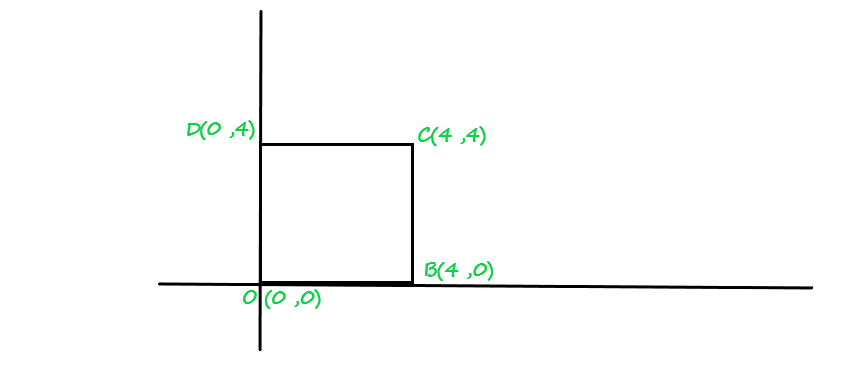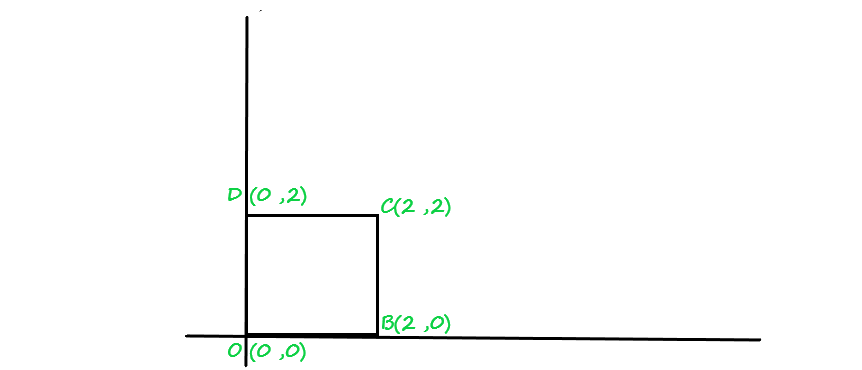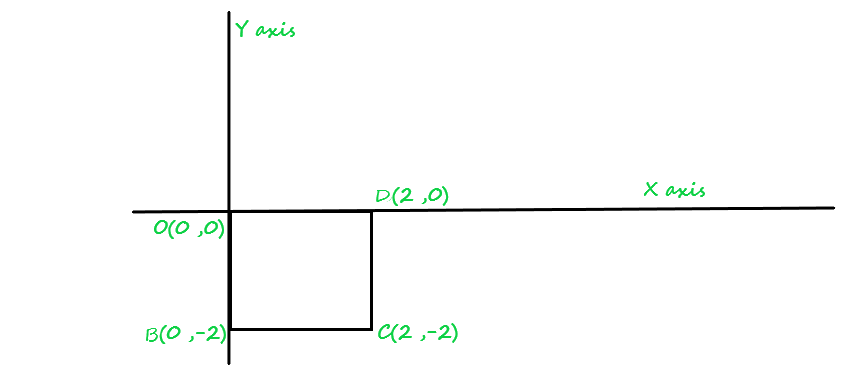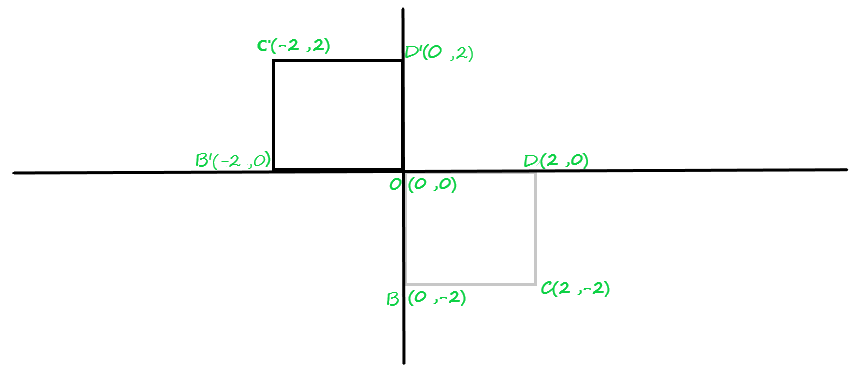GeeksforGeeks App
Open AppBrowser
Continue

# Composite Transformation in 2-D graphics

Prerequisite – Basic types of 2-D Transformation :

Composite Transformation :
As the name suggests itself Composition, here we combine two or more transformations into one single transformation that is equivalent to the transformations that are performed one after one over a 2-D object.

Example :
Consider we have a 2-D object on which we first apply transformation T1 (2-D matrix condition) and then we apply transformation T2(2-D matrix condition) over the 2-D object and the object get transformed, the very equivalent effect over the 2-D object we can obtain by multiplying T1 & T2 (2-D matrix conditions) with each other and then applying the T12 (resultant of T1 X T2) with the coordinates of the 2-D image to get the transformed final image.

Problem :
Consider we have a square O(0, 0), B(4, 0), C(4, 4), D(0, 4) on which we first apply T1(scaling transformation) given scaling factor is Sx=Sy=0.5 and then we apply T2(rotation transformation in clockwise direction) it by 90*(angle), in last we perform T3(reflection transformation about origin).

Ans : The square O, A, C, D looks like :Square_given(Fig.1)

First, we perform scaling transformation over a 2-D object :

Representation of scaling condition :For coordinate O(0, 0) :For coordinate B(4, 0) :For coordinate C(4, 4) :For coordinate D(0, 4) :2-D object after scaling :Fig.2

*Now, we’ll perform rotation transformation in clockwise-direction on Fig.2 by 90θ:

The condition of rotation transformation of 2-D object about origin is :For coordinate O(0, 0) :For coordinate B(2, 0) :For coordinate C(2, 2) :For coordinate D(0, 2) :2-D object after rotating about origin by 90* angle :Fig.3

*Now, we’ll perform third last operation on Fig.3, by reflecting it about origin :

The condition of reflecting an object about origin isFor coordinate O(0, 0) :For coordinate B'(0, 0) :For coordinate C'(0, 0) :For coordinate D'(0, 0) :The final 2-D object after reflecting about origin, we get :Fig.4

Note : The above finale result of Fig.4, that we get after applying all transformation one after one in a serial manner. We could also get the same result by combining all the transformation 2-D matrix conditions together and multiplying each other and get a resultant of multiplication(R). Then, applying that 2D-resultant matrix(R) at each coordinate of the given square(above). So, you will get the same result as you have in Fig.4.

Solution using Composite transformation :
*First we multiplied 2-D matrix conditions of Scaling transformation with Rotation transformation :*Now, we multiplied Resultant 2-D matrix(R1) with the third last given Reflecting condition of transformation(R2) to get Resultant(R) :Now, we’ll applied the Resultant(R) of 2d-matrix at each coordinate of the given object (square) to get the final transformed or modified object.

First transformed coordinate O(0, 0) is :Second, transformed coordinate B'(4, 0) is :Third transformed coordinate C'(4, 4) is :Fourth transformed coordinate D'(0, 4) is :The final result of the transformed object that you get would be same as above :My Personal Notes arrow_drop_up